# Prime factors

Write 98 as a product of prime factors

Result

f = (Correct answer is: 2 * 7 * 7)### Step-by-step explanation: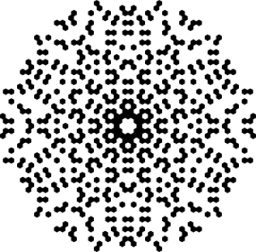Did you find an error or inaccuracy? Feel free to write us. Thank you!

Showing 1 comment:## Related math problems and questions:The heights of five starters on redwood high’s basketball team are 5’11”, 6’3”, 6’6”, 6’2” and 6’. The average of height of these players is?
• Sum of fractionsWhat is the sum of 2/3+3/5?
• Median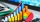The number of missed hours was recorded in 11 pupils: 5,12,6,8,10,7,5,110,2,5,6. Determine the median.
• LCD two fractionsWhat is the least common denominator of 11/15 and 12/19?
• Simplify 3Simplify mixed numerals expression: 8 1/4- 3 2/5 - (2 1/3 - 1/4) Show your solution.
• Prime factorsFactor the number 6600 into the product of prime numbers.
• Decomposition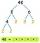Make decomposition using prime numbers of number 155. Result write as prime factors (all, even multiple)
• Mixed numbersRewrite mixed numbers, so the fractions have the same denominator: 5 1/5 - 2 2/3
• Turtles 2A box turtle hibernates in the sand at 11 5/8. A spotted turtle hibernates at 11 16/25 feet. Which turtle is deeper? Write answer as number 1 or 2.
• Two forcesThe two forces F1 = 580N and F2 = 630N, have an angle of 59 degrees. Calculate their resultant force, F.
• Linear combination of complex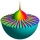If z1=5+3i and z2=4-2i, write the following in the form a+bi a) 4z1+6z2 b) z1*z2
• Tiles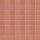How many 46 cm square tiles will cover a floor 22.08 m by 8.74 m?
• Evaluate 5Evaluate expression x2−7x+12x−4 when x=−1
• BoatsThree-quarters of boats are white, 1/7 are blue and 9 are red. How many boats do we have?
• Write 2Write 791 thousandths as fraction in expanded form.
• Emily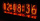Emily had 20 minutes to do a three-problem quiz. She spent 11 3/4 minutes on question A and 5 1/2 minutes on question B. How much time did she have left for question C?
• Solve 2Solve integer equation: a +b+c =30 a, b, c = can be odd natural number from this set (1,3,5,7,9,11,13,15)